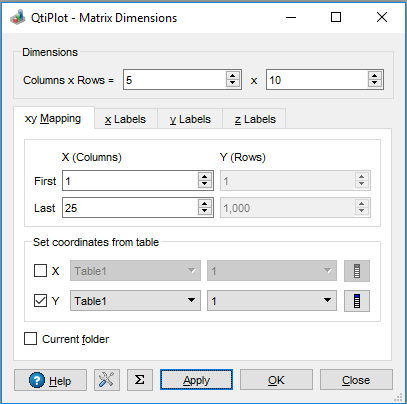Set Matrix Dimensions

This command appears in the Matrix menu. It allows direct specification of the number of rows and columns of a matrix. In this window, you can also define a linear range for X-values and Y-values. These X and Y ranges will be used in new plots as the initial scale ranges. They are also available via the x and y variables if you choose to define the contents of the matrix with the Set Values Dialog.

Figure 5-145. The Set Dimensions... dialog for matrix.It is also possible to define non-linear X/Y coordinates using data values from table columns via the controls in the Set coordinates from table group box. If the Current folder box is checked only the tables from the current project subfolder are displayed in the table selection list boxes.

The column used for the X coordinates must contain a number of valid, non empty cells at least equal with the number of columns in the matrix. Similarly, the column used for the Y coordinates must contain a number of valid, non empty cells at least equal with the number of rows in the matrix. QtiPlot sorts these data values in ascending order before assigning them as matrix coordinates.

You can also define a Long Name, Units and Comments for X / Y / Z coordinates under the X Labels, Y Labels or Z Labels tab. These labels can be used as X / Y /Z axis labels in 3D graphs.